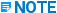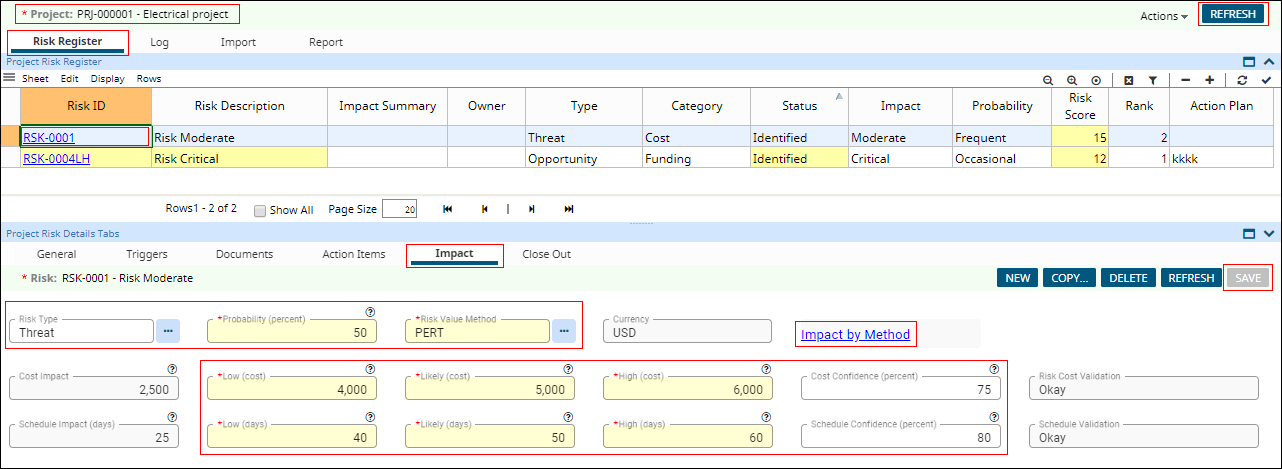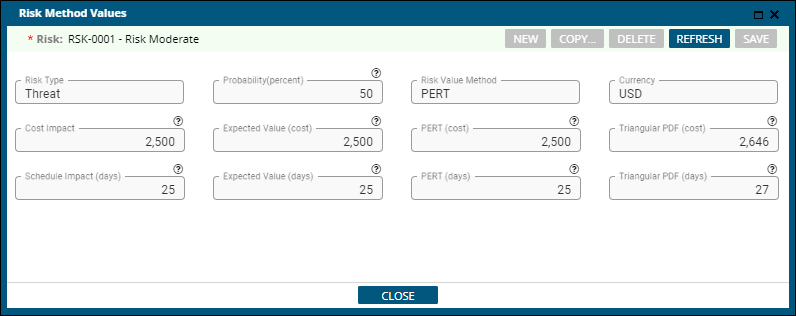Calculate Risk Impact - EcoSys - 3.01 - Help - Hexagon PPM

EcoSys Projects Help (3.01)

Language
English (United States)
Product
EcoSys
Search by Category
Help
EcoSys Standard Version
3.01

Risk Impact calculates the quantitative cost and schedule impact of a risk. A risk value method is used to determine the risk impact for both cost and schedule.

The risk methods are:

• Expected Value

• PERT

• Triangular PDF

Expected Value

This method determines the value that a risk will contribute to the overall project risk value based on the impact value and the probability of occurrence.

PERT

This is an industry standard method to determine the normalized value that a risk will contribute to the overall project risk value based on the weighted values (inputs for low, high, and likely) and the probability of occurrence.

Triangular PDF

This is an industry standard method to determine the value that a risk will contribute to the overall project risk value. This method uses the value for a specific confidence level on the probability density function for a triangular distribution (inputs for low, high, and likely) and the probability of occurrence.

The risk impact value is positive for threats and negative for opportunities. The user input fields are set to mandatory based on the method selected.

This following procedure allows you to calculate the impact of the risk using Risk Value Method.

1. In the Project Risk Details Tabs panel, click the Impact tab. The existing action items appear.

2. Click the Risk Type ellipsis to change the risk type.

3. Type in the Probability (percent).

4. Click the Risk value Method ellipsis to select the method to calculate the risk.According to the selected method, the mandatory fields to be filled in change.

5. Type in the below values:

1. Low (cost)

2. Likely (cost)

3. High (cost)

4. Low (days)

5. Likely (days)

6. High (days)Enter the values for Low, High, and Likely (cost/days) according to the selected method.

6. Type in the percentages below:

1. Cost Confidence (percent)

2. Schedule Confidence (percent)

7. Click SAVE.8. Click the Impact by Method hyperlink. The calculated risk impact values appear in the Risk Method Values dialog.